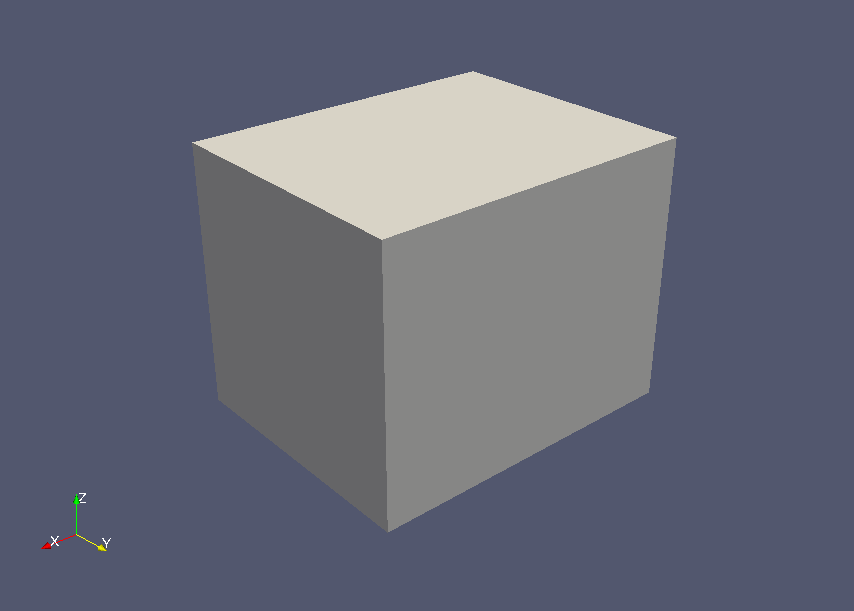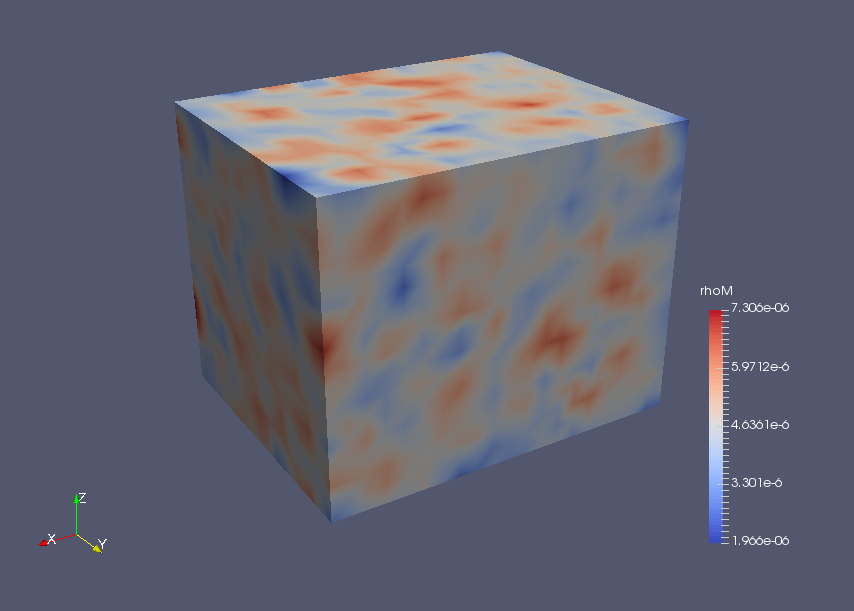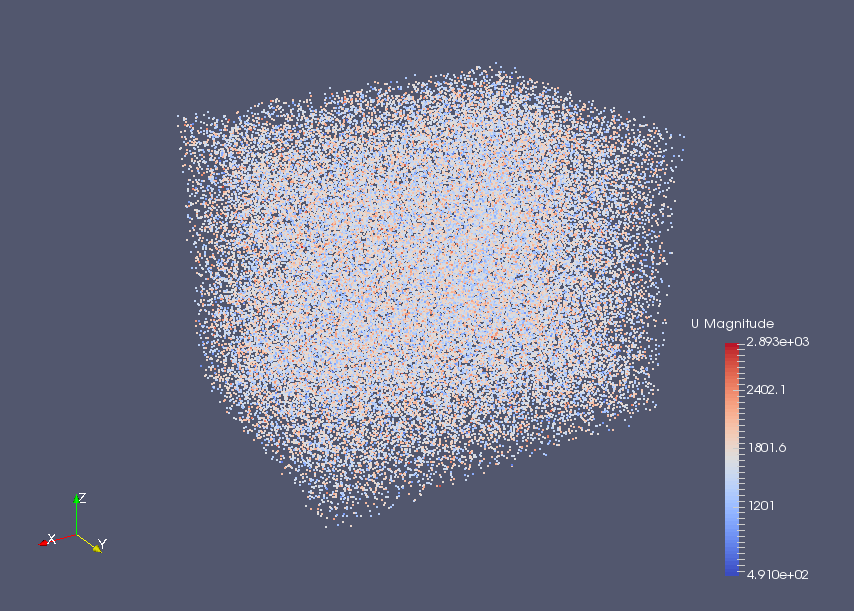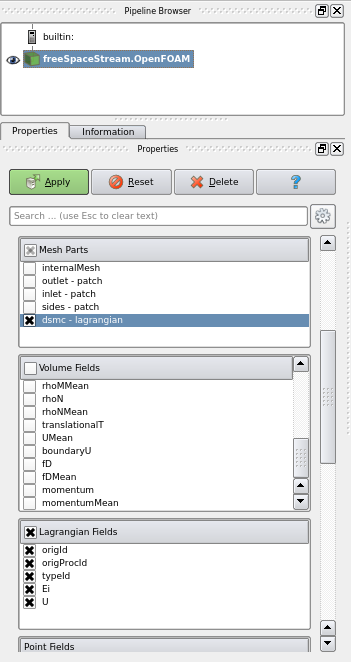﻿ Direct simulation Monte Carlo of oxygen/nitrogen mixtures (periodic boundary) - XSim

Direct simulation and Monte Carlo simulation of oxygen/nitrogen mixtures (periodic boundary)

Update: January 3, 2018
OpenFOAM 4.x

Case directory

\$FOAM_TUTORIALS/discreteMethods/dsmcFoam/freeSpacePeriodic

Summary

We calculate the mixture gas flow of oxygen and nitrogen with the Direct Simulation Monte Carlo (DSMC) method. The Direct Simulation Monte Carlo (DSMC) method was proposed by G. A. Bird in the 1960s to calculate the flow of rarefied gases using the Boltzmann equation.

The properties of the gas are defined in the file "dsmcProperties" in the directory "constant", and the initial state is defined in the file "dsmcInitialiseDict" in the directory "system".

The size of the model in the X, Y, and Z axis directions is 10 cm, 8 cm, and 8 cm, respectively, and each direction faces are periodic boundary conditions.Model geometry

Each variable in directory 0 is as follows. The X, Y, and Z axis directions of the model are periodic boundary conditions, respectively.

• boundaryT - Boundary temperature
• boundaryU - Boundary velocity
• dsmcRhoN - DSMC particles Density
• fD - Force Density (stress vector)
• q - Wall heat flux
• iDof - Density of internal degrees of freedom
• internalE - Internal energy density
• linearKE - Linear kinetic energy density
• momentum - Momentum density
• rhoM - Mass density
• rhoN - Number density
• dsmcSigmaTcRMax - maximum value of "sigmaT × cR" in each cell (see Bird p220)

The calculation result is as follows.Mass density at final timeDSMC particles at final time

Visualization operations

To visualize DSMC particles, load the data into ParaView by the paraFoam command, and then set the following in the Properties tab of the pipeline browser and click the Apply button.

• Check only "dsmc" in the Mesh Parts list
• Uncheck all items in Volume Fields list
• Check all items in Lagrangian Fields listSelect display items

Commands

cp -r \$FOAM_TUTORIALS/discreteMethods/dsmcFoam/freeSpacePeriodic freeSpacePeriodic
cd freeSpacePeriodic

blockMesh
dsmcInitialise
dsmcFoam

paraFoam

Calculation time

60.2 seconds *Single, Inter(R) Core(TM) i7-2600 CPU @ 3.40GHz 3.40GHz